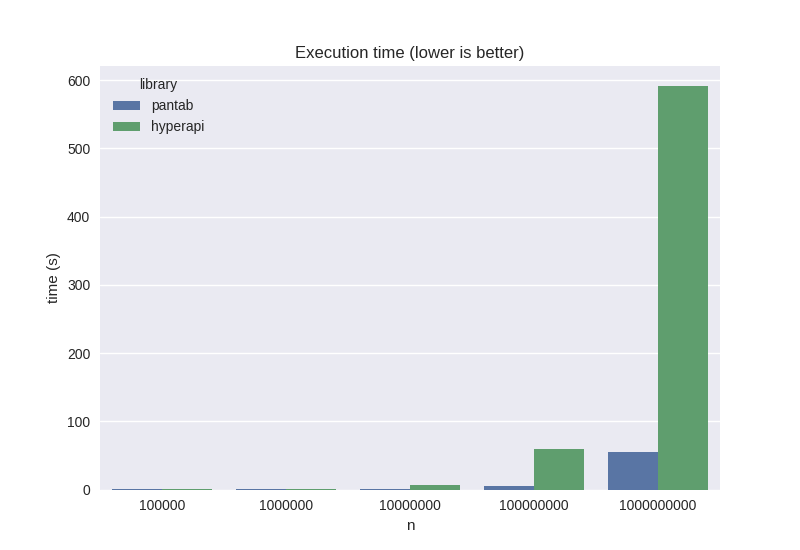# pantab¶

Important

pantab is currently incompatible with tableauhyperapi>=0.0.14567. For details and status updates see GitHub issue #145

## What is it?¶

`pantab` is a Python wrapper around Tableau’s Hyper API which promotes usage of the pandas DataFrame to seamlessly generate and read hyper extracts.

## How do I get it?¶

`pantab` requires Python 3.6+ and can run on any Python-supported OS. Installation is as easy as:

```python -m pip install pantab
```

## Why should I use it?¶

`pantab` is faster than what you would write by hand, in less lines of code. Consider the below benchmark:This was generated from the output of the below code on Manjaro Linux with 4.6 GHz CPU and 16 GB of memory.

```import itertools
import time

import numpy as np
import pandas as pd
import pantab
from tableauhyperapi import (
Connection,
CreateMode,
HyperProcess,
Inserter,
SqlType,
TableDefinition,
TableName,
Telemetry,
)

def write_via_pantab(proc: HyperProcess, n: int):
df = pd.DataFrame({"column": np.ones(n, dtype=np.int16)})
pantab.frame_to_hyper(df, "example.hyper", table="table", hyper_process=proc)

def write_via_hyperapi(proc: HyperProcess, n: int):
data_to_insert = np.ones(n, dtype=np.int16)

table = TableDefinition(
table_name=TableName("table"),
columns=[TableDefinition.Column(name="column", type=SqlType.int())],
)

with Connection(
endpoint=proc.endpoint,
database="example.hyper",
create_mode=CreateMode.CREATE_AND_REPLACE,
) as conn:
conn.catalog.create_table(table)

with Inserter(conn, table) as inserter:
for data in data_to_insert:
inserter.execute()

ns = (100_000, 1_000_000, 10_000_000, 100_000_000, 1_000_000_000)
funcs = (write_via_pantab, write_via_hyperapi)
for n, func in itertools.product(ns, funcs):
with HyperProcess(telemetry=Telemetry.DO_NOT_SEND_USAGE_DATA_TO_TABLEAU) as hyper:
start = time.time()
func(hyper, n)
end = time.time()
print(
f"Total seconds for function {func.__name__} with {n} rows: {end - start}"
)
```

Running this script may yield the below

```Total seconds for function write_via_pantab with 100_000 rows: 0.026696205139160156
Total seconds for function write_via_hyperapi with 100000 rows: 0.08622312545776367
Total seconds for function write_via_pantab with 1000000 rows: 0.10160708427429199
Total seconds for function write_via_hyperapi with 1000000 rows: 0.6384274959564209
Total seconds for function write_via_pantab with 10000000 rows: 0.7043006420135498
Total seconds for function write_via_hyperapi with 10000000 rows: 6.226941108703613
Total seconds for function write_via_pantab with 100_000_000 rows: 5.718722820281982
Total seconds for function write_via_hyperapi with 100000000 rows: 59.03396558761597
Total seconds for function write_via_pantab with 1000000000 rows: 54.43922758102417
Total seconds for function write_via_hyperapi with 1000000000 rows: 591.2542216777802
```